Download Presentation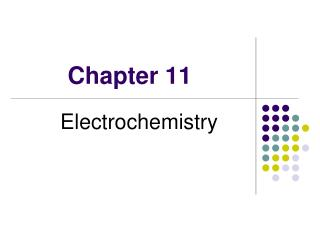Chapter 11

# Chapter 11 - PowerPoint PPT Presentation

Chapter 11. Electrochemistry. Electromotive Force ( 電動勢 ). 1 joule of work is produced or required when 1 coulomb of charge is transferred between two points in the circuit that differ by a potential of 1 volt. Galvanic Cells (Voltaic Cells ).I am the owner, or an agent authorized to act on behalf of the owner, of the copyrighted work described.
Download Presentation## Chapter 11

An Image/Link below is provided (as is) to download presentation

Download Policy: Content on the Website is provided to you AS IS for your information and personal use and may not be sold / licensed / shared on other websites without getting consent from its author.While downloading, if for some reason you are not able to download a presentation, the publisher may have deleted the file from their server.

- - - - - - - - - - - - - - - - - - - - - - - - - - E N D - - - - - - - - - - - - - - - - - - - - - - - - - -
Presentation Transcript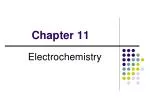### Chapter 11

Electrochemistry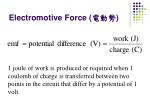Electromotive Force (電動勢)

1 joule of work is produced or required when 1 coulomb of charge is transferred between two points in the circuit that differ by a potential of 1 volt.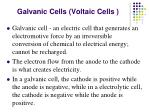Galvanic Cells (Voltaic Cells )
• Galvanic cell - an electric cell that generates an electromotive force by an irreversible conversion of chemical to electrical energy; cannot be recharged.
• The electron flow from the anode to the cathode is what creates electricity.
• In a galvanic cell, the cathode is positive while the anode is negative, while in a electrolytic cell, the cathode is negative while the anode is positive.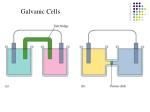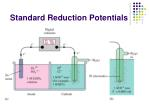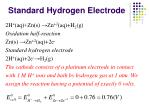Standard Hydrogen Electrode

2H+(aq)+Zn(s) →Zn+2(aq)+H2(g)

Oxidation half-reaction

Zn(s) →Zn+2(aq)+2e-

Standard hydrogen electrode

2H+(aq)+2e-→H2(g)

The cathode consists of a platinum electrode in contact

with 1 M H+ ions and bath by hydrogen gas at 1 atm. We

assign the reaction having a potential of exactly 0 volts.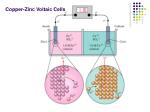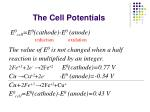The Cell Potentials

E0cell=E0(cathode)-E0 (anode)

The value of E0 is not changed when a half

reaction is multiplied by an integer.

2Fe+3+2e-→2Fe+2E0(cathode)=0.77 V

Cu →Cu+2+2e- -E0 (anode)=-0.34 V

Cu+2Fe+3 →2Fe+2+Cu+

E0cell=E0(cathode)-E0 (anode)=0.43 V

reduction

oxidationCell Diagrams

For a copper-zinc voltaic cells

Cu’ Zn ZnSO4(aq) CuSO4(aq) Cu

1. A vertical line indicates a phase boundary.

2. A dashed vertical line indicates the phase boundary between two miscible liquid.

Dashed linePtL|H2(g)|HCl(aq)|AgCl(s)|Ag| PtR

Anode: H2(g)=2H++2e-(PtL)

Cathode: [AgCl(s)+e-(PtR)=Ag+Cl-] ×2

Overall: 2AgCl(s)+ H2(g)=2Ag+ 2H++2Cl-

CuL|Cd(s)|CdCl2(0.1M)|AgCl(s)|Ag(s) |CuR

Anode: Cd=Cd+2+2e-

Cathode: [AgCl+e-=Ag++Cl-] ×2

Overall: Cd+2AgCl=2Ag+Cd+2+2Cl-Nernst Equation
• E0: standard reduction potential
• n: moles of electrons
• F: Faraday constant 96485 C/molThermodynamic-Free Energy
• The maximum cell potential is directly related to the free energy difference between the reactants and the products in the cell.Reaction Quotient (Q)

The positive E (fR>fL ) means that Q<K0. As Q

increases toward K0, the cell emf decreases,

reaching zero when Q=K0The Equilibrium Constant of a Cu-Zn Cell

Zn+Cu+2(aq)=Zn+2(aq)+Cu

E0=0.34V-(-0.76V)=1.10VAgL Ag+(0.1M) Ag+(1M) AgR

PtL Cl2(PL) HCl(aq) Cl2(PR) PtRLiquid Junction Potential
• Liquid junction: the interface between two miscible electrolyte solutions.
• Liquid-junction potential: A potential difference between two solutions of different compositions separated by a membrane type separator.
• The salt will diffuse from the higher concentration side to the lower concentration side.H+

H+

Cl-

Ag+

HCl HCl

AgNO3 HNO3

a2 < a1

a2 = a1Liquid Junction Potential
• The diffusion rate of the cation and the anion of the salt will very seldom be exactly the same.
• Assume the cations move faster; consequently, an excess positive charge will accumulate on the low concentration side, while an excess negative charge will accumulate on the high concentration side of the junction due to the slow moving anions.
• When the cell has a liquid junction, the observed cell emf includes the additional potential difference between the two electrolyte solutions.How to Solve the Liquid Junction Potential
• Liquid junction potentials are generally small, but they certainly cannot be neglected in accurate work.
• By connecting the two electrolyte solutions with a salt bridge, the junction potential can be minimized.
• A salt bridge consist of a gel made by adding agar to a concentrated aqueous KCl solution.A Cell Diagrams Containing a Salt Bridge

For a copper-zinc voltaic cells

Cu’ Zn ZnSO4(aq) CuSO4(aq) Cu

A salt bridge is symbolized by two vertical dashed lines.Estimate the Liquid Junction Potential from EMF Measurement

Ag AgCl(s) LiCl(m) NaCl(m) AgCl(s) Ag

m(LiCl)=m(NaCl), E0=0

Anode: Ag+Cl- (in LiCl(aq))=AgCl+e-

Cathode: AgCl+e-=Ag+Cl- (in NaCl(aq))Applications of Electrochemistry
• pH meter
• ATP Synthase
• Potential for a resting nerve cellDetermination of pH

Pt H2(g) soln. X KCl(sat.) Hg2Cl2(s) Hg Pt’

1/2H2(g)+1/2Hg2Cl2=Hg(l)+H+(aq,X)+Cl-(aq)

The cell reaction and emf Ex:

Junction potential between X and the saturated KCl solutionIf a second cell is set up to except that solution

X is replaced by solution S, the emf Es of this

cell will be:Reference electrode:

saturated calomel electrode (SCE)

Sensing electrode:

Ion Selective Electrode (ISE)

Pt Ag AgCl(s) HCl(aq) glass soln. X KCl(sat.) Hg2Cl2(s) Hg Pt’Determine the pH of a Solution by a pH Meter
• When the glass electrode is immersed in solution X, an equilibrium between H+ ions in solution and H+ ions in the glass surface is set up.
• This charge transfer between glass and solution produces a potential difference between the glass and solution.Ion Selective Electrodes (ISE) for PH Meter
• An ion selective electrode contains a glass, crystalline, or liquid membrane whose nature is such that the potential difference between the membrane and an electrolyte solution it is in contact with is determined by the activity of one particular ion.
• It is dependent on the concentration of an ionic species in the test solution and is used for electro-analysis.Marcus theory for Electron transfer reactions

• Rudolph A. Marcus was awarded the 1992 Nobel Prize in chemistryMembrane Equilibrium

In a closed electrochemical system, the phase equilibrium condition for two phases a and bFree-energy change during proton movement across a concentration gradient
• The movement of protons from the cytoplasm into the matrix of the mitochondrion.Proton Pumping
• Proton pumping maintains a pH gradient of 1.4 units, then DpH = + 1.4
• DG = -2.303RTΔpH

=- 2.303 (8.315 × 10-3 kJ/mol)(298K)(1.4)

= - 7.99 kJ/mol• In mitochondria, electron transport drives proton pumping from the matrix into the intermembrane space.
• There is no compensating movement of other charged ions, so pumping creates both a concentration gradient and a voltage gradient.
• This voltage component makes the proton gradient an even more powerful energy source.Membrane Potential
• Dym = yin – yout=0.14 V
• DG =-nF Dym=-(1)(96485)(0.14 ) = - 13.5 kJ/molProton-motive force
• Proton-motive force (DP) is a Dy that combines the concentration and voltage effects of a proton gradient.
• DG=-nFDP = - 2.303 RT DpH + nFDym

=(-7.99 kJ/mol)+( - 13.5 kJ/mol)

= -21.5 kJ/molATP synthesis
• Mitochondrial proton gradient as a source of energy for ATP synthesis
• Estimated consumption of the proton gradient by ATP synthesis is about 3 moles protons per mole ATP.
• DG = 50 kJ/mol for ATP synthesis
• DG = 50 + 3(- 21.5) = - 14.5 kJ/mol
• The synthesis of ATP is spontaneous under mitochondrial conditions.P: permeability (穿透率)

D: diffusion coefficient (擴散係數)

t: thickness of membrane (薄膜厚度)Concentrations cell

△E(K+)=-95 mV

△E(Na+)=+57 mV

△E(Cl-)=-67 mV

Resting Nerve Cell of a Squid

P(K+) /P(Cl-)=2

P(K+)/P(Na+)=25

The observed potential for a resting squid nerve cell is about

-70 mV at 25oC.Resting Nerve Cell of a Squid
• The observed potential for a resting squid nerve cell is about -70 mV at 25oC.
• Hence Cl- is in electrochemical equilibrium, but K+ and Na+ are not.
• Na+ continuously flows spontaneously into the cell and K+ flows spontaneously out.
• Na+-K+ pumpBatteries
• Secondary batteries: Voltaic cells whose electrochemical reactions can be reversed by a current of electrons running through the battery after the discharge of an electrical current.
• A secondary battery can be restored to nearly the same voltage after a power discharge.Anode reaction

Pb+HSO4-→ PbSO4+H++2e-

Cathode reaction

PbO2+HSO4-+3H++2e- → PbSO4+2H2O

Cell reaction

Pb+PbO2+ 2H++2HSO4-→ 2PbSO4+2H2ODry Cell Battery

Anode reaction

Zn→ Zn2++2e-

Cathode reaction

2NH4++2MnO2+2e- → Mn2O3+2NH3+H2O

Cell reaction

2MnO2+2NH4Cl+Zn→ Zn(NH3)2Cl2 + Mn2O3

+H2OAlkaline Dry Cell

Anode reaction

Zn+2OH- → ZnO+H2O+2e-

Cathode reaction

MnO2+2H2O+2e- → Mn2O3+2OH-

Cell reaction

MnO2+H2O+Zn→ Mn2O3+ZnOAnode reaction

2H2+4OH-→4H2O+4e-

Cathode reaction

4e-+O2+2H2O →4OH-

Cell reaction

2H2+O2 →2H2OAnodic Region

Fe→Fe+2+2e-

Cathodic Region

O2+2H2O+4e- →4OH-

Overall Reaction

4Fe+2(aq)+O2(g)+(4+2n) H2O(l)

→2Fe2O3‧nH2O(s)+8H+(aq)Electrolysis
• Electrolytic Cell: use electrical energy to produce chemical change
• The process of electrolysis involves forcing a current through a cell to produce a chemical change for which the cell potential is negative.Zn+Cu+2→Zn+2+Cu Zn+2+Cu→Zn+Cu+2Electrolysis of Water

Anode reaction: 2H2O→O2+4H++4e-

Cathode reaction: 4H2O+4e-→2H2+4OH-

2H2O →2H2+O2E0=-2.06VElectrolysis of Mixture of Ions
• A solution in an electrolytic cell contains the ions Cu+2, Ag+ and Zn+2.
• The more positive the E0 value, the more the reaction has a tendency to proceed in the direction indicated.
• Ag+ > Cu+2 > Zn+2Electrolysis of NaCl/H2O System

Anode reaction:

2H2O→O2+4H++4e- -E0=-1.23 V

2Cl-→Cl2+2e--E0=-1.36 V

The Cl- ion is first to be oxidized. A much higher

potential than expected is required to oxidized

water. The voltage required in excess of the

excepted (overvoltage) is much greater for the

production of O2 than for Cl2.